Skip to main content

# Semi-periodic solutions of difference and differential equations

## Abstract

The spaces of semi-periodic sequences and functions are examined in the relationship to the closely related notions of almost-periodicity, quasi-periodicity and periodicity. Besides the main theorems, several illustrative examples of this type are supplied. As an application, the existence and uniqueness results are formulated for semi-periodic solutions of quasi-linear difference and differential equations.

MSC:34C15, 34C27, 34K14, 39A10, 42A16, 42A75.

## Introduction

In , it is observed that although the set of periodic sequences forms a linear space, its uniform closure is not the space of almost-periodic sequences but of semi-periodic sequences. In fact, the space of semi-periodic sequences was shown there to be Banach.

The whole Sections I.6, I.7 in  and Sections II.4, II.5 in  are devoted to semi-periodic continuous functions, called there limit periodic functions (cf. also [, p.129]). This class was shown there to be identical with the one of uniformly almost-periodic functions with one-term -base and, in case of integral one-term base, it reduces to the one of purely periodic functions. For some more references concerning limit periodic functions, see, e.g., [5, 6]. In fact, limit periodic functions were already considered by Bohr in 1925, as pointed out in [, p.113].

In the following section, we define analogously to  the class of semi-periodic continuous functions (with values in a Banach space) and show that it is the same as the class of limit periodic functions considered in [2, 3] (see Theorem 1 below). Let us note that many different notions with the same name (i.e., semi-periodic), like functions satisfying Floquet boundary conditions (see, e.g., [7, 8]) or those describing Bloch waves (see, e.g., , and the references therein), exist in the literature (cf. also [9, 10]).

Hence, after giving a definition of semi-periodic functions, which is analogous to , we prove that the uniform closure of the set of periodic functions is again the one of semi-periodic functions. Unlike in the discrete case, the space of semi-periodic functions is, however, not linear and so not Banach. In order to clarify transparently the position of semi-periodic sequences and functions in the hierarchy of closely related spaces, we decided to illustrate it by means of Venn’s diagrams. Thus, the spaces of almost-periodic, semi-periodic, quasi-periodic and periodic functions and sequences and some of their sums (in the continuous case) are compared in this way. For this, the semi-periodicity is considered by means of the Fourier-Bohr coefficients.

There are even more general interesting classes of almost-periodic functions (for their hierarchy, see, e.g., [11, 12]), but for our needs here only those which are uniformly (Bohr) a.p. will be taken into account. It is well known that uniformly continuous Stepanov a.p. functions are Bohr a.p. (see, e.g., [4, 11]). Another nontraditional characterization of Bohr almost-periodicity was recently done in , namely that Stepanov a.p. functions with Stepanov a.p. derivatives are also Bohr a.p.

In order to make applications to difference and differential equations, we still need to define the notion of uniform semi-periodicity and prove that the associated Nemystkii operators map the set of semi-periodic sequences into themselves. This is unfortunately not true in the case of functions. On this basis, we finally give two examples about the existence of semi-periodic solutions in the form of theorems, both in the discrete and in the continuous cases. Although many various sorts of periodic-type solutions were investigated (for their panorama, see ), as far as we know, semi-periodic solutions in the sense of definitions below of difference or differential equations have been only considered in  and in a certain sense also in . Nevertheless, as pointed out in , Johnson  and Millionshchikov  have already given examples of limit periodic differential equations which admit almost automorphic solutions, but not limit periodic ones.

Before passing to semi-periodic functions in the next section, it will be convenient to mention some facts about semi-periodic sequences.

Hence, denoting as usually by $(N)$ the set of (positive) integers and letting $E$ to be a Banach space endowed with the norm $| ⋅ | E$, let us recall the definition of semi-periodic sequences (cf. ).

Definition 1 A sequence $x ̲ ∈ E Z$ is called semi-periodic (s.p.) if

$∀ε>0,∃T∈N,∀n∈Z,∀k∈Z, | x k + n T − x k | E ≤ε.$

One can readily check that Definition 1 can be regarded as a discrete version of Definition 2 below for semi-periodic functions. Similarly, the definition of quasi-periodic (q.p.) sequences can be regarded as a discretized (i.e., restricted to ) version of the one for quasi-periodic functions recalled below. A q.p. extending function has the Fourier-Bohr expansion with $Mod(⋅)$ to be finitely generated which is also true for q.p. sequences. For more properties and details concerning q.p. functions, see, e.g., .

In this light, since the analogy of Theorem 2 below holds for sequences (see Remark 4) and since the discrete (i.e., restricted to ) analogies of Examples 1-3 below can be constructed, one can illustrate the relationship of these classes by means of Venn’s diagram in Figure 1. For more properties about s.p. sequences, see, e.g., [1, 18, 19].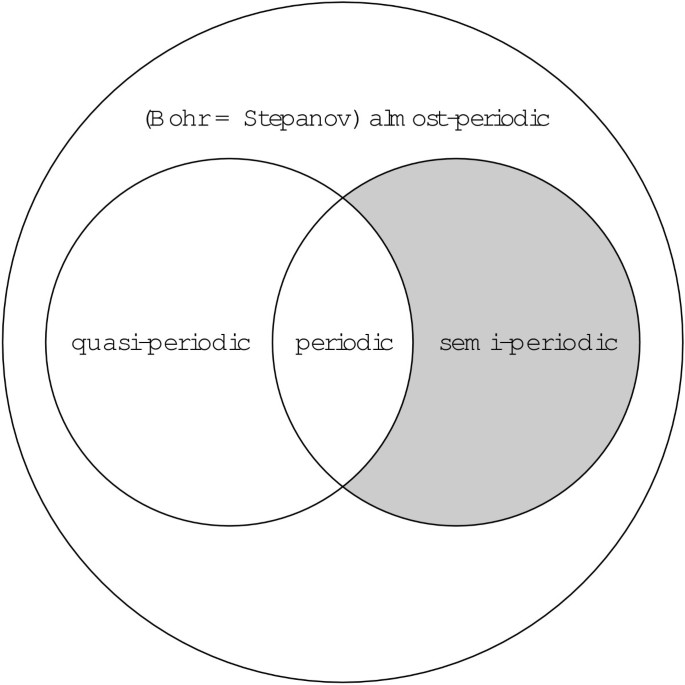Figure 1

On the other hand, the situation in Figure 1 is much simpler than in Figure 2 for continuous functions, because under the restriction to , the sum of (semi-)periodic sequences remains (semi-)periodic while Stepanov almost-periodic (a.p.) sequences were shown in  to coincide with Bohr a.p. sequences.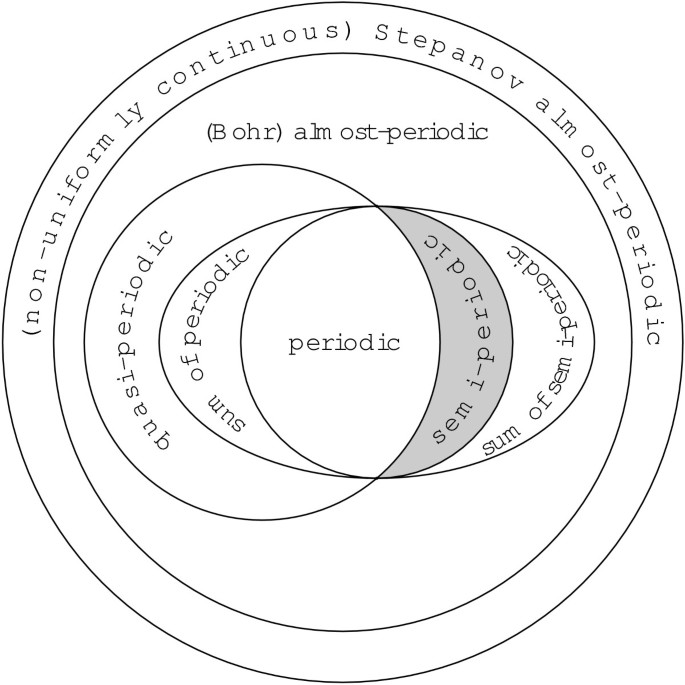Figure 2

## Continuous semi-periodic functions

Let $C T 0 (R,E)$ be the set of continuous T-periodic functions,

$Per(R,E):= ⋃ T > 0 C T 0 (R,E)$

be the set of periodic functions and $BC 0 (R,E)$ be the set of continuous bounded functions. The last one is a Banach space with the uniform norm (written $∥ ⋅ ∥ ∞$).

Definition 2 A continuous function $f∈ C 0 (R,E)$ is said to be semi-periodic (s.p.) if

$∀ε>0,∃T>0,∀n∈Z,∀t∈R, | f ( t + n T ) − f ( t ) | E ≤ε.$

Such a T will be called an ε-semi-period of f.

Let $S(R,E)$ denote the set of semi-periodic functions.

It is easy to see from the definition that every continuous periodic function is semi-periodic. Moreover, if f is semi-periodic, then f is uniformly (Bohr) almost-periodic (i.e., $f∈ AP 0 (R,E)$), and so it is bounded. Thus, we can rewrite Definition 2 as follows.

Definition 3 A (bounded) continuous function $f∈ C 0 (R,E)$ is said to be semi-periodic (s.p.) if

$∀ε>0,∃T>0,∀n∈Z, ∥ f ( ⋅ + n T ) − f ( ⋅ ) ∥ ∞ ≤ε.$

We have

$Per(R,E)⊂S(R,E)⊂ AP 0 (R,E)⊂ BC 0 (R,E).$

From this, we can consider $S(R,E)$ as a metric space, when using

$d(f,g):= sup t ∈ R | f ( t ) − g ( t ) | E .$

As we will see later, $S(R,E)$ is not a linear space, but $S(R,E)$ is a complete metric space.

Lemma 1 Let $f∈S(R,E)$, $ε>0$ and $T ε$ be an ε-semi-period of f. Then there exists a continuous $T ε$-periodic function φ s.t.

$∥ f − φ ∥ ∞ ≤ε.$

Proof Consider a $T ε$-periodic function ψ such that its restriction to $[0; T ε )$ is the same as the one of f. For each $x∈R$, we can write $x=t+n T ε$ with $n∈Z$ and $t∈[0; T ε )$. Thus, we get

$| f ( x ) − ψ ( x ) | E = | f ( t + n T ε ) − ψ ( t + n T ε ) | E = | f ( t + n T ε ) − ψ ( t ) | E = | f ( t + n T ε ) − f ( t ) | E ≤ ε .$

Since ψ is not necessarily continuous, consider still $τ∈(0; T ε )$ such that, for any $t∈[ T ε −τ, T ε ]$, $| f ( t ) − f ( T ε ) | E ≤ε$. Define a $T ε$-periodic continuous function φ which is equal to ψ on $[0, T ε −τ]$ and which is linear on $[ T ε −τ, T ε ]$. For $t∈[ T ε −τ, T ε ]$, we obtain

$| f ( t ) − φ ( t ) | E ≤ T ε − t τ | f ( T ε − τ ) − f ( t ) | E + t − ( T ε − τ ) τ | f ( T ε ) − f ( t ) | E ≤ 2 ε ,$

and subsequently

$sup x ∈ R | φ ( x ) − f ( x ) | E ≤2ε.$

□

Remark 1 For $E=R$, unlike for semi-periodic functions in the sense of Definition 2 or Definition 3, in fact the same lemma was already proved in [, pp.114-115], but for limit periodic functions. As already pointed out in the foregoing section, these classes will be shown to coincide by Theorem 1 below, whose proof is just based on Lemma 1.

We are ready to give the first theorem.

Theorem 1 $S(R,E)$ is the closure of $Per(R,E)$ in the sup-norm.

Proof Assume firstly that f is s.p. Taking in Lemma 1 $ε n =1/n$, we obtain a sequence of periodic functions $( φ n ) n$ s.t. $∥ f − φ n ∥ ∞ ≤ ε n →0$.

Reversely, assume that f is in the closure of the set of continuous T-periodic functions. Then, for any $ε>0$, we can find a periodic φ s.t. $| f ( t ) − φ ( t ) | E ≤ε$. Let T be its period. Then, for any $t∈R$,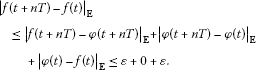□

Remark 2 In view of Theorem 1, one can now also define a semi-periodic function, equivalently w.r.t. Definition 2 and Definition 3, as the uniform limit of a uniformly convergent sequence of continuous purely periodic functions. This was so done, e.g., in [2, 3, 5, 6, 14].

In the following proposition, we look for the link between s.p. sequences and functions. Given a sequence $x ̲ = ( x t ) t ∈ Z$, we set $f x ̲ :R→E$, the function s.t. its restriction to is $x ̲$ and which is linear on each $[k,k+1]$, $k∈Z$, i.e.,

$∀t∈Z, f x ̲ (u):={u} x t + 1 + ( 1 − { u } ) x t ,$

where ${u}$ is the fractional part of u, i.e., ${u}∈[0,1)$ and $u−{u}∈Z$.

Proposition 1 Let $x ̲ ∈ E Z$. All the following statements are equivalent:

1. 1.

$f x ̲$ is s.p. with a semi-period in ,

2. 2.

there exists a s.p. function with a semi-period in whose restriction to is $x ̲$,

3. 3.

$x ̲$ is s.p.

Proof For (1) (2), take $f x ̲$ in (2). For (2) (3), take T as an ε-semi-period for the function f in (2). Then we have

$∀ t ∈ Z , | x t + T − x t | E = | f ( t + T ) − f ( t ) | E ≤ ∥ f ( ⋅ + T ) − f ∥ ∞ ≤ ε .$

For (3) (1), given T as an ε-semi-period of $x ̲$, we have for all $t∈Z$□

Let us now consider the Fourier expansion of a semi-periodic function. Recall that every a.p. function has the Fourier-Bohr expansion,

$f(t)∼ ∑ j = 1 ∞ a λ j (f) e i λ j t ,$

where

$a λ (f):=M { f ( t ) e − i λ t } ,$

and

$M{g}:= lim l → ∞ ( 2 l ) − 1 ∫ − l l g(t)dt$

is the mean operator (see, e.g., [3, 4, 11]). It follows from the above formula that $f↦ a λ (f)$ is 1-Lipschizian (and so it is continuous) from $AP 0 (R,E)$ to $E$.

Set $Λ(f):={λ, a λ (f)≠0}$ and denote by $Mod(f)$ the -modulus generated by $Λ(f)$. Recall that an a.p. function is quasi-periodic (q.p.) if $Mod(f)$ has a finite -basis, and that T is a period of f if and only if $Λ(f)⊂ 2 π T Z$ (see, e.g., [4, 17]).

Proposition 2 (for $E=R$, cf. [, p.32])

Set $Q:={ r n ,n∈N}$ and consider

$f(t):= ∑ λ ∈ θ Q a λ (f) e i λ t ,$

for a fixed $θ>0$, where

$∑ λ ∈ θ Q | a λ ( f ) | E <+∞.$

Then f is s.p.

Proof Consider

$f N (t)= ∑ n = 1 N a r n θ (f) e i r n θ t .$

Clearly, if $r n = p n q n$, then $2 π q n p n θ$ is a period of the n th term. The same is obviously true for $2 π q n θ$. Thus, $2 π q 1 ⋯ q N θ$ is a period of $f N$ which is so periodic. Moreover,

$∥ f − f N ∥ ∞ ≤ ∑ n ≥ N + 1 | a r n θ ( f ) | E →0,$

which already proves that f is s.p. □

The following result is also, at least for $E=R$, well known (see, e.g., , [, pp.118-119], and the references therein).

Lemma 2 If $f∈S(R,E)$, then there exists $θ>0$ s.t.

$Λ(f)⊂θQ.$

Proof Let us consider λ and μ s.t. $a λ (f)≠0$ and $a μ (f)≠0$ and a sequence of periodic functions $( f n ) n$ s.t. $f n →f$, uniformly. It follows from the continuity that, for sufficiently large N, $a λ ( f N )≠0$ and $a μ ( f N )≠0$, but since $f N$ is periodic, it follows that $λ/μ∈Q$. □

Remark 3

1. 1.

This proof also demonstrates that, for a sufficiently large n, the period $T n$ of $f n$ satisfies $T n θ∈2πQ$.

2. 2.

It indicates that $S(R,E)$ is not a linear space. For instance, a simple q.p. function $t↦cos(t)+cos(t 2 )$ is not s.p. although it is a sum of two s.p. functions. On the other hand, the sum of two a.p. functions is trivially a.p.

Example 1 On the basis of Proposition 2 and Lemma 2, we can easily give the following example of a purely s.p. (i.e., not periodic) function:

$f(t)= ∑ n ≥ 1 e i t / n n 2 .$

Moreover, one can readily check that the function f can be obtained as a uniform limit of the sequence $( f N ) N$, where $f N$ is a continuous $2πN!$-periodic function,

$f N (t)= ∑ n = 1 N e i t / n n 2 .$

Theorem 2 Every s.p. function which is also q.p. is in fact periodic:

$S(R,E)∩ QP 0 (R,E)=Per(R,E).$

Proof Let $f∈S(R,E)∩ QP 0 (R,E)$. Since f is q.p., we can find $ω 1 ,…, ω m$ such that

$Λ(f)⊂Z ω 1 +⋯+Z ω m .$

Set $G 1 :=Z ω 1 +⋯+Z ω m$. $G 1$ is an additive subgroup of . Since $f∈S(R,E)$, we can find $θ>0$ s.t. $Λ(f)⊂θQ$. Set $G 2 :=θQ$. $G 2$ is another additive subgroup of , so $G= G 1 ∩ G 2$ is a subgroup of $G 1$ which contains $Λ(f)$. Since G is a subgroup of $G 1$, there exist $p∈{1,…,m}$ and positive -independent real numbers $ζ 1 ,…, ζ p$ s.t.

$G=Z ζ 1 +⋯+Z ζ p .$

Let us show that $p=1$. Once we have it, we can conclude that $Λ(f)⊂ ζ 1 Z$ which proves that $2 π ζ 1$ is a period of f. Since, for each i, $ζ i ∈G⊂ G 2$, we know that, for each i, we can find $q i ∈Q$ s.t. $ζ i = q i θ$. This proves that $ζ i / ζ j ∈Q$, for $i≠j$, which is impossible. □

Remark 4 In view of Proposition 1 and its analogy for q.p. sequences mentioned in the foregoing section, a discrete (i.e., restricted to ) analogy of Theorem 2 holds for sequences.

Example 2 As an example of a function which is almost-periodic (a.p.) but neither quasi-periodic nor a sum of semi-periodic functions, consider

$f(t)= ∑ n ≥ 1 e i σ n t n 2 ,$

where the $σ k$’s are constructed by induction, say for all k,

$σ k + 1 ∉ σ 1 Q+⋯+ σ k Q.$

We will prove that we cannot find a finite set of numbers $θ 1 ,…, θ q$ s.t.

$Λ(f)⊂ θ 1 Q+⋯+ θ q Q.$

Firstly, assume this has already been proved. Then if f is a sum of semi-periodic functions $f j$, say $f= ∑ j = 1 q f j$, we could find, according to Lemma 2, for each j a $θ j$ s.t. $Λ( f j )⊂ θ j Q$. This implies that

$Λ(f)⊂Λ( f 1 )∪⋯∪Λ( f q )⊂( θ 1 Q)+⋯+( θ q Q),$

which is not true. If f were quasi-periodic, we could find $θ 1 ,…, θ q$ s.t.

$Λ(f)⊂ θ 1 Z+⋯+ θ q Z⊂ θ 1 Q+⋯+ θ q Q,$

which is again wrong. Now, we can make the first part of the proof. So, let us assume

$Λ(f)⊂ θ 1 Q+⋯+ θ q Q.$

We have $Λ(f)={ σ i ,i≥1}$. Thus, for any $i≥1$, we can find $( a i 1 ,…, a i q )∈ Q q ∖{0}$ s.t.

$σ i = ∑ j = 1 q a i j θ j .$

Let us now consider the square matrix

$A= ( a i j ) 1 ≤ i , j ≤ q .$

If it is invertible, we can express $θ 1 ,…, θ q$ linearly (with rational coefficients) depending on $σ 1 ,…, σ q$. This proves that $σ q + 1$ should be a (rational) linear combination of $σ 1 ,…, σ q$, which is not true.

Assuming that the matrix is singular, its rows are linearly dependent. So, we can find $( μ 1 ,…, μ q )∈ Q q ∖{0}$ s.t. $∑ i μ i a i j =0$, for each j. Multiplying it by $θ j$ and then summing over j, we obtain $∑ i μ i σ i =0$ which is not possible.

Example 3 As an example of a function which is quasi-periodic (q.p.) but not a sum of periodic functions, consider

$f(t)= ∑ n ≥ 1 e i t ( 1 + n 2 ) n 2 .$

Here $Λ(f)={1+n 2 ,n∈N}$, thus $Mod(f)=Z+ 2 Z$, i.e., f is q.p. Assume that f is a sum of a finite number of periodic functions. Let $T 1 ,…, T k$ be the periods. According to , we have

$Δ( T 1 ,…, T k )f=0,$

where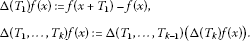An easy calculation yields

$a λ ( Δ ( T 1 , … , T k ) f ) = a λ (f) ∏ j = 1 k ( e i λ T j − 1 ) ,$

by which

$∀n∈N,∃j∈{1,…,k},(1+n 2 ) T j ∈2πZ.$

Since is infinite, we can find two different integers m, n with the same $T j$. Thus, there exist two integers $k m$, $k n$ s.t.

$1 + n 2 k n = 2 π T j = 1 + m 2 k m .$

This implies that $k m ≠ k n$, and we obtain

$( k m − k n )=(m k n −n k m ) 2 ,$

which is not possible.

Remark 5 We know (see, e.g., [4, 11]) that every almost-periodic (a.p.) f is a uniform limit of a sequence of a finite sum of periodic functions $( f n ) n$. Writing

$f= f 0 + ∑ n ( f n + 1 − f n ),$

we can see that every a.p. function can be expressed as a series of periodic functions. Reversely, a uniformly convergent series of periodic functions is a.p.

Summing up the above observations, we can present in Figure 2 Venn’s diagram for continuous functions under our investigation. The classes of almost-periodic, semi-periodic and quasi-periodic functions are in circles, while sums of semi-periodic functions are in the ellipse. Sums of periodic functions are in the intersection of the classes of quasi-periodic functions and sums of semi-periodic functions. In fact, one can check by similar arguments as in the proof of Theorem 2 that a sum of periodic functions is exactly the sum of semi-periodic functions which is quasi-periodic. Periodic functions are, according to Theorem 2, at the same time semi-periodic and quasi-periodic. Purely semi-periodic functions are in the grey strip.

Now, consider the primitives of s.p. functions.

Lemma 3 Assume that f is a.p. and consider $F(t):= ∫ 0 t f(s)ds$. Assume that there exists $φ∈ AP 0 (R,E)$ and $a∈E$ s.t.,

$∀t∈R,F(t)=φ(t)+at.$

Then $a=M{f}$.

Indeed, φ is necessarily differentiable, and integrating the equality $f= φ ′ +a$, we obtain

$a= 1 2 l ∫ − l l f(s)ds+O ( 1 l ) ,$

because φ is bounded. This already proves Lemma 3. It is well known that $M{f}=0$ is a necessary and sufficient condition for F to be periodic, provided f is so. It is, however, not sufficient in the case of a.p. functions. For more details, see, e.g., . Despite the approximation by periodic functions, it is also not sufficient in the case of s.p. functions, as demonstrated by the following example.

Example 4 Let us consider the s.p. function

$f(t)= ∑ n ≥ 1 cos ( t / n 2 ) n 2 .$

We have a normal convergence, so the series exists and defines a s.p. function for which $M{f}=0$. A formal candidate to be its primitive is

$F(t)= ∑ n ≥ 1 sin ( t / n 2 ) .$

We have a uniform convergence on each compact set, because $|sin(u)|≤|u|$. Thus, this series also exists and defines a primitive of f. If F were s.p., it should be a.p. which is obviously not true, because the Parseval equality does not apply.

## Uniformly semi-periodic functions with respect to a parameter

Definition 4 Let $f:R×M→ R k$, where M is a subset of $R n$. We say that f is uniformly semi-periodic (u.s.p.) if for any compact set $K⊂M⊂ R n$, we have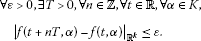Since such a function is u.a.p., we know that given a compact subset K of M, f is bounded and uniformly continuous on $R×K$.

Proposition 3 Any u.s.p. function is a uniform limit, on each $R×K$, of a sequence of continuous functions which are periodic w.r.t. their first variables.

Proof Let T be given by the definition and consider a T-periodic function $φ(⋅,α)$ such that its restriction to $[0;T)$ is the same as the one of $f(⋅,α)$. For each $x∈R$, we can write $x=t+nT$ with $n∈Z$ and $t∈[0;T)$. Thus, we get

$| f ( x , α ) − φ ( x , α ) | R k = | f ( t + n T , α ) − φ ( t + n T , α ) | R k = | f ( t + n T , α ) − φ ( t , α ) | R k = | f ( t + n T , α ) − f ( t , α ) | R k ≤ ε ,$

uniformly w.r.t. $α∈K$. Since φ is not necessarily continuous, consider still $τ∈(0;T)$ such that, for any $t∈[T−τ,T]$ and any $α∈K$, $| f ( t , α ) − f ( T , α ) | E ≤ε$. This is possible, because K is compact. Define a T-periodic continuous function $ψ(⋅,α)$ which is equal to $φ(⋅,α)$ on $[0,T−τ]$ and which is linear on $[T−τ,T]$. For $t∈[T−τ,T]$, we obtain

$| f ( t , α ) − ψ ( t , α ) | R k ≤ T − t τ | f ( T − τ , α ) − f ( t , α ) | R k + t − ( T − τ ) τ | f ( T , α ) − f ( t , α ) | R k ≤ 2 ε ,$

and subsequently

$sup ( x , α ) ∈ R × K | ψ ( x , α ) − f ( x , α ) | R k ≤2ε.$

□

Remark 6 Assume that f is L-Lipschitzian w.r.t. its second variable. It follows from the proof that so is φ, from which we can deduce the same for ψ. So, a u.s.p. function Lipschitzian w.r.t. its second variable can be approximated uniformly on each $R×K$ (K compact) by a sequence of functions which are periodic w.r.t. their first variables and Lipschitzian (with the same constant L) w.r.t. their second variables.

Remark 7 It is possible to define the same for the discrete case and to obtain analogous results. This will be omitted here, because the proofs are quite similar.

Concerning the Nemytskii operator, in the continuous case, it is not true that if f is u.s.p. and ϕ is s.p., then $t↦f(t,ϕ(t))$ is s.p. As an example, take $f(t,x)=sin(t)+x$ and $ϕ(t)=sin(πt)$. On the other hand, it is true in the discrete case.

Proposition 4 Assume that $f:Z×M→ R p$ is s.p. and that $x ̲ = ( x t ) t$ is s.p. with the range in $M⊂ R n$. Then the sequence $( f ( t , x t ) ) t ∈ Z$ is s.p.

Proof Set $K= { x t , t ∈ Z } ¯$. Since $x ̲$ is a.p., K is a compact subset of M. So, given $ε>0$, we can find $η>0$ s.t.

$sup t ∈ Z , | x − y | ≤ η | f ( t , x ) − f ( t , y ) | R p ≤ε.$

Set $η ′ :=min{η,ε}$. We know that we can find two integers $T 1$, $T 2$ s.t.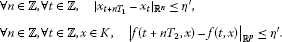Let T be a common multiplier of $T 1$ and $T 2$ (for instance, $T= T 1 T 2$). The last inequalities remain true, when replacing every $T i$ by T. Thus, for any $(t,n)∈ Z 2$,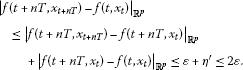□

For an alternative proof, one can employ the approximation by periodic sequences.

## Semi-periodic solutions of difference equations

In this section, we are interested in semi-periodic solutions of the difference equation in $R p$,

$x t + 1 +A x t =f(t, x t ),$
(1)

where A is a real square $p×p$ matrix.

Theorem 3 Assuming that A has no eigenvalues with modulus one and that f is u.s.p. and Lipschitzian w.r.t. the second variable with a sufficiently small constant, there exists a unique semi-periodic solution for the difference equation (1).

Proof We know (see, e.g., Proposition 2.2 in ) that, for each a.p. sequence $( b t ) t$ with values in $R p$, there exists a unique a.p. solution to

$x t + 1 +A x t = b t .$
(2)

Denoting by $AP(Z, R p )$ the Banach space of a.p. sequences (cf. ), the linear operator $T:AP(Z, R p )→AP(Z, R p )$, determined by the left-hand side of (2), is obviously invertible. Since T is continuous satisfying $∥T∥≤1+∥A∥$, we know from the well-known Banach theorem that $T − 1$ must be continuous as well.

Now, consider a s.p. sequence $( q t ) t$ with values in $R p$. We are firstly interested in the a.p. solution to the equation

$x t + 1 +A x t =f(t, q t ).$
(3)

By the hypothesis imposed on f and in view of Proposition 4, $( f ( t , q t ) ) t$ is s.p. Therefore, there exists a unique a.p. solution of (3) (see again Proposition 2.2 in ). We can now consider $T − 1 ( ( f ( t , q t ) ) t )$. Since $T − 1$ maps the space of periodic sequences into itself, by the unique solvability of (3) in $AP(Z, R n )$ and by the continuity of $T − 1$, the mapping

$T: ( q t ) t → T − 1 ( ( f ( t , q t ) ) t )$

maps $S(Z, R p )$ into itself. Denote by L the Lipschitz constant to all $f(t,⋅)$. It is easy to see that $∥ T − 1 ∥L$ is a Lipschitz constant for $T$.

Assuming that $L<1/∥ T − 1 ∥$, the mapping T is a contraction in the Banach space $S(Z, R p )$. So it has a unique fixed point representing the desired s.p. solution of (1). □

Remark 8 Using a triangular form of −A (like Jordan’s one) (see, e.g., [, Proposition 6.14 and Remark 6.26]), it is possible to compute explicitly a constant c s.t. $∥ T − 1 ∥≤c$. For such a constant, it is sufficient to assume $L<1/c$ in order to justify Theorem 3.

## Semi-periodic solutions of differential equations

Let us consider the equation

$x ′ +Ax=f(t,x).$
(4)

We assume that a real square $k×k$ matrix A has an exponential dichotomy property, i.e., that there exist a projection matrix P ($P= P 2$) and constants $C>0$, $λ>0$, such that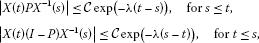where X is the fundamental matrix of $x ′ +Ax=0$ satisfying $X(0)=I$, i.e., the unit matrix (see, e.g., [, Chapter III.5]). Furthermore, let $f:R× R k → R k$ be u.s.p. with respect to the variable x.

Setting

$C(A):= sup t ∈ R | ∫ R | G ( t − s ) | ds|≤ sup t ∈ R ∫ R C e − λ | t − s | ds= 2 C λ ,$

where

is the Green function associated to A, and $P −$, $P +$ stand for the corresponding spectral projections on the invariant subspaces of A, we can formulate the following theorem.

Theorem 4 Assume still that f is L-Lipschitzian w.r.t. the second variable with $L<(λ/2C≤)1/C(A)$. Then there exists a unique semi-periodic solution of the equation (4).

Proof Let $( f n ) n$ be a sequence of periodic functions w.r.t. their first variables s.t. $f n →f$, uniformly. We can assume without any loss of generality (see Remark 3) that each $f n$ is L-Lipschitzian w.r.t. its second variable. Let $x n$ be the unique bounded (in fact, periodic) solution of the equation

$x ′ +Ax= f n (t,x)$

and $x ¯$ be the unique bounded solution of (4). Such solutions exist; for more details, see, e.g., [, Chapter III.5].

It will be sufficient to show that $x n → x ¯$, uniformly.

We have the integral representations (see again, e.g., [, Chapter III.5])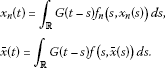It can be easily checked that, in view of uniqueness of bounded solutions, the periods $T n$ of $f n$ are also periods of $x n$. It holds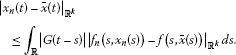Now, let us prove that there exists a uniform estimate to all $x n$. We have

$| x n ( t ) | R k ≤ ∫ R | G ( t − s ) | | f n ( s , x n ( s ) ) | R k ds,$

and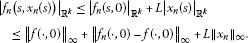Thus,

$∥ x n ∥ ∞ ≤C(A) ( ∥ f ( ⋅ , 0 ) ∥ ∞ + ∥ f n ( ⋅ , 0 ) − f ( ⋅ , 0 ) ∥ ∞ + L ∥ x n ∥ ∞ )$

and, according to $C(A)L<1$, still

$∥ x n ∥ ∞ ≤ C ( A ) 1 − C ( A ) L ( ∥ f ( ⋅ , 0 ) ∥ ∞ + ∥ f n ( ⋅ , 0 ) − f ( ⋅ , 0 ) ∥ ∞ ) =:R,$

where R is the desired bound. Putting $K= B ( 0 , R ) ¯$, we arrive at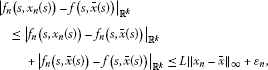where $ε n = sup R × K ∥ f n −f∥→0$. Thus, we finally get

$∥ x n − x ¯ ∥ ∞ ≤ C ( A ) 1 − C ( A ) L ε n →0.$

Since $x n$ are $T n$-periodic, we conclude that $x ¯$ is semi-periodic. □

## Concluding remarks

Remark 9 Because of the right-hand side $f(t,x)$ in (4), even in the scalar case, Theorem 4 cannot be deduced from the results in , where the scalar equation $x ′ +g(x)=f(t)$ was considered.

Remark 10 Since Theorem 3 and Theorem 4 represent only illustrative examples, the obtained existence and uniqueness criteria were tendentiously very simple. More sophisticated situations will be considered by ourselves elsewhere.

Remark 11 Analogously as in [24, 25], where almost-periodic solutions were under consideration, it would be interesting to obtain similar results concerning semi-periodic solutions of monotone systems or those treated by means of variational methods.

## References

1. 1.

Berg JD, Wilansky A: Periodic, almost-periodic, and semiperiodic sequences. Mich. Math. J. 1962, 9: 363-368.

2. 2.

Besicovitch AS: Almost Periodic Functions. Dover, New York; 1954.

3. 3.

Levitan BM: Almost-Periodic Functions. GITTL, Moscow; 1953. in Russian

4. 4.

Corduneanu C: Almost Periodic Oscillations and Waves. Springer, Berlin; 2009.

5. 5.

Bell H, Meyer KR: Limit periodic functions, adding machines, and solenoids. J. Dyn. Differ. Equ. 1995, 7: 409-422. 10.1007/BF02219369

6. 6.

Schwarz W, Spilker J: Arithmetical Functions. Cambridge University Press, Cambridge; 1994.

7. 7.

Andres J: Periodic-type solutions of differential inclusions. 8. In Advances in Mathematical Research. Edited by: Baswell AR. Nova Sciences Publishers, New York; 2009:295-353.

8. 8.

Andres J, Górniewicz L: Topological Fixed Point Principles for Boundary Value Problems. Kluwer Academic, Dordrecht; 2003.

9. 9.

Ichihara N, Ishii H: Asymptotic solutions of Hamilton-Jacobi equations with semi-periodic Hamiltonians. Commun. Partial Differ. Equ. 2008, 33: 784-807. 10.1080/03605300701257427

10. 10.

Lorenz EN: Noisy periodicity and reverse bifurcation. Annals of the New York Academy of Sciences 357. Nonlinear Dynamics 1980, 282-291.

11. 11.

Andres J, Bersani AM, Grande RF: Hierarchy of almost-periodic function spaces. Rend. Mat. Appl. 2006, 26: 121-188.

12. 12.

Corduneanu C: A scale of almost periodic function spaces. Differ. Integral Equ. 2011, 24: 1-28.

13. 13.

Andres J, Pennequin D: On the nonexistence of purely Stepanov almost-periodic solutions of ordinary differential equations. Proc. Am. Math. Soc. 2012, 140: 2825-2834. 10.1090/S0002-9939-2012-11154-2

14. 14.

Alonso AI, Obaya R, Ortega R: Differential equations with limit-periodic forcings. Proc. Am. Math. Soc. 2002, 131: 851-857.

15. 15.

Johnson RA: On almost-periodic linear differential systems of Milionshchikov and Vinograd. J. Math. Anal. Appl. 1982, 85: 452-460. 10.1016/0022-247X(82)90011-7

16. 16.

Millionshchikov VM: Proof of the existence of irregular systems of linear differential equations with almost periodic coefficients. Differ. Equ. 1968, 4: 203-205.

17. 17.

Blot J, Pennequin D: Spaces of quasi-periodic functions and oscillations in differential equations. Acta Appl. Math. 2001, 65: 83-113. 10.1023/A:1010631520978

18. 18.

Goes G: Fourier-Stieltjes transforms of discrete measures; periodic and semiperiodic functions. Math. Ann. 1967, 174: 148-156. 10.1007/BF01360815

19. 19.

Jiménez MN: Multipliers on the space of semiperiodic sequences. Trans. Am. Math. Soc. 1985, 291: 801-811.

20. 20.

Andres J, Pennequin D: On Stepanov almost-periodic oscillations and their discretizations. J. Differ. Equ. Appl. 2012, 18: 1665-1682. 10.1080/10236198.2011.587813

21. 21.

Mortola S, Peirone R: The sum of periodic functions. Boll. Unione Mat. Ital. 1999, 8: 393-396.

22. 22.

Andres J, Bednařík D, Pastor K: On the notion of derivo-periodicity. J. Math. Anal. Appl. 2005, 303: 405-417. 10.1016/j.jmaa.2004.08.020

23. 23.

Pennequin D: Existence of almost periodic solutions of discrete time equations. Discrete Contin. Dyn. Syst. 2001, 7: 51-60.

24. 24.

Blot J, Cieutat P, Mawhin J: Almost periodic oscillations of monotone second-order systems. Adv. Differ. Equ. 1997, 2: 693-714.

25. 25.

Mawhin J: Bounded and almost periodic solutions of nonlinear differential equations: variational vs nonvariational approach. Res. Notes Math. 410. In Calculus of Variations and Differential Equations. Edited by: Ioffe A, Reich S, Shafrir I. Chapman & Hall/CRC, Boca Raton; 1999:167-184.

Download references

## Acknowledgements

The first author was supported by the project A-Math-Net Applied Mathematics Knowledge Transfer Network No CZ.1.07/2.4.00/17.0100.

## Author information

Authors

### Corresponding author

Correspondence to Jan Andres.

## Additional information

### Competing interests

The authors declare that they have no competing interests.

### Authors’ contributions

All authors contributed equally in this article. They read and approved the final manuscript.

## Authors’ original submitted files for images

Below are the links to the authors’ original submitted files for images.

## Rights and permissions

Open Access This article is distributed under the terms of the Creative Commons Attribution 2.0 International License (https://creativecommons.org/licenses/by/2.0), which permits unrestricted use, distribution, and reproduction in any medium, provided the original work is properly cited.

Reprints and Permissions

## About this article

### Cite this article

Andres, J., Pennequin, D. Semi-periodic solutions of difference and differential equations. Bound Value Probl 2012, 141 (2012). https://doi.org/10.1186/1687-2770-2012-141

Download citation

• Received:

• Accepted:

• Published:

### Keywords

• semi-periodic sequences
• semi-periodic functions
• semi-periodic solutions
• difference equations
• differential equations